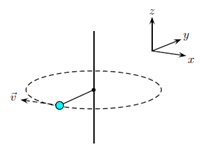Problem: A person spins a tennis ball on a string in a horizontal circle in the x−y plane as shown in the image. The ball is moving clockwise when viewed from above. Just when the string is parallel with the y-axis, the ball is hit with a force in the direction of the ball’s velocity. This causes a change in the ball’s angular momentum ΔL in the1. The angular momentum does not change (zero torque).2. +z direction3. −z direction4. +x direction5. −x direction6. +y direction7. −y direction

95% (95 ratings)
Problem Details

A person spins a tennis ball on a string in a horizontal circle in the x−y plane as shown in the image. The ball is moving clockwise when viewed from above. Just when the string is parallel with the y-axis, the ball is hit with a force in the direction of the ball’s velocity. This causes a change in the ball’s angular momentum ΔL in the

1. The angular momentum does not change (zero torque).

2. +z direction

3. −z direction

4. +x direction

5. −x direction

6. +y direction

7. −y direction